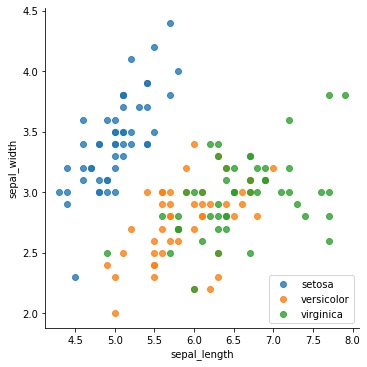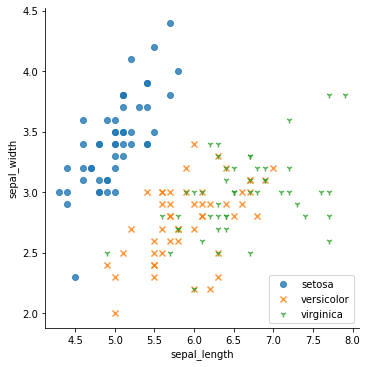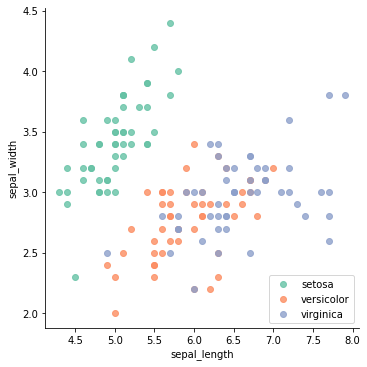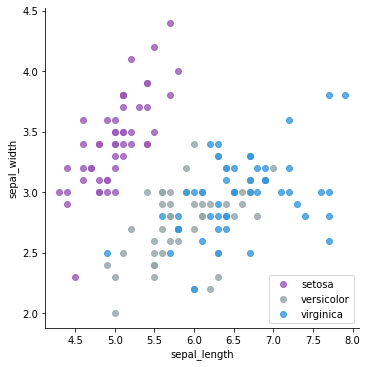# Use categorical variable to color scatterplot in seaborn

In this post, you will see how to use hue argument in a basic scatterplot in order to define groups in your data by different colors or shapes.

Using seaborn library, you can plot a basic scatterplot with the ability to use color encoding for different subsets of data. In the following examples, the iris dataset from seaborn repository is used. Using `hue` argument, it is possible to define groups in your data by different colors or shapes.

## Map a color per group

This example uses `lmplot()` function of seaborn library. In order to define each species with different colors, species column of the dataset given in `hue` argument. The list of arguments needed for the function is:

• `x` : positions of points on the X axis
• `y` : positions of points on the Y axis
• `data` : dataset
• `fit_reg` : if True, show the linear regression fit line
• `hue` : variables that define subsets of the data
• `legend` : if True, add a legend

Note that the legend is specified in through matplotlib, instead of seaborn itself. In order to specifically define a location of the legend, `plt.legend()` can be used.

``````# library & dataset
import seaborn as sns
import matplotlib.pyplot as plt

# Use the 'hue' argument to provide a factor variable
sns.lmplot( x="sepal_length", y="sepal_width", data=df, fit_reg=False, hue='species', legend=False)

# Move the legend to an empty part of the plot
plt.legend(loc='lower right')

plt.show()``````## Map a marker per group

It is also possible to define categories with different marker shapes. You can do it by giving `markers` argument to the function:

• `markers` : a list of marker shapes
``````# library & dataset
import seaborn as sns
import matplotlib.pyplot as plt

# give a list to the marker argument
sns.lmplot( x="sepal_length", y="sepal_width", data=df, fit_reg=False, hue='species', legend=False, markers=["o", "x", "1"])

# Move the legend to an empty part of the plot
plt.legend(loc='lower right')

plt.show()``````## Use another palette

Instead of using default color pallette, you can specify your pallette choice by `palette` parameter. There are many palettes available in seaborn including deep, muted, bright, pastel, dark, and colorblind.

``````# library & dataset
import seaborn as sns
import matplotlib.pyplot as plt

# Use the 'palette' argument
sns.lmplot( x="sepal_length", y="sepal_width", data=df, fit_reg=False, hue='species', legend=False, palette="Set2")

# Move the legend to an empty part of the plot
plt.legend(loc='lower right')

plt.show()``````## Control color of each group

Another alternative to specify a color palette for dataset groups in a seaborn scatterplot is creating a dictionary mapping hue levels to matplotlib colors.

``````# library & dataset
import seaborn as sns
import matplotlib.pyplot as plt

# Provide a dictionary to the palette argument
sns.lmplot( x="sepal_length", y="sepal_width", data=df, fit_reg=False, hue='species', legend=False, palette=dict(setosa="#9b59b6", virginica="#3498db", versicolor="#95a5a6"))

# Move the legend to an empty part of the plot
plt.legend(loc='lower right')

plt.show()``````## Contact & Edit

👋 This document is a work by Yan Holtz. Any feedback is highly encouraged. You can fill an issue on Github, drop me a message onTwitter, or send an email pasting `yan.holtz.data` with `gmail.com`.